Next: 3.5 Mathematical Induction Up: 3. Propositions and Functions Previous: 3.3 Functions   Index

# 3.4 Summation Notation

Let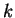and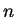be integers with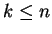. Let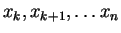, be real numbers, indexed by the integers fromto. We define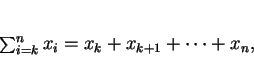(3.53)

i.e.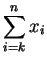is the sum of all the numbers. A sum of one number is defined to be that number, so that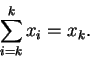The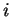'' in equation (3.53) is a dummy variable, and can be replaced by any symbol that has no meaning assigned to it. Thus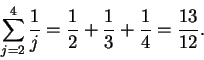The following properties of the summation notation should be clear from the definition. (Here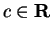,andare integers withand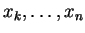and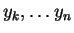are real numbers.)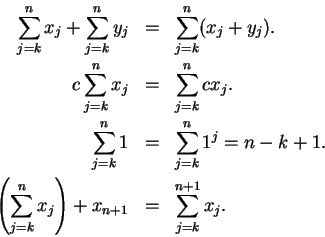If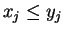for all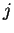satisfying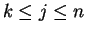then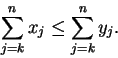Also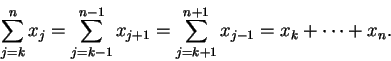Using the summation notation, we can rewrite equations (2.9) and (2.23) as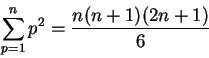and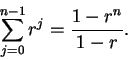The use of the Greek letter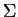to denote sums was introduced by Euler in 1755[15, page 61]. Euler writes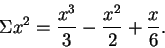Compare this with the notation in Bernoulli's table 2.2. (The apparent difference is due to the fact that for Euler,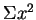denotes the sum of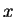squares, starting with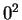, whereas for Bernoulli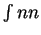denotes the sum ofsquares starting with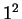.) The use of the symbol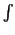(which is a form of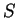) for sums was introduced by Leibniz. The use of limits on sums was introduced by Augustin Cauchy(1789-1857). Cauchy used the notation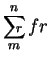to denote what we would write as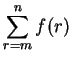[15, page 61].

3.54   Exercise. Find the following sums:

a)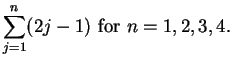b)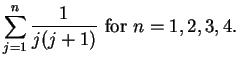c)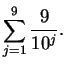Next: 3.5 Mathematical Induction Up: 3. Propositions and Functions Previous: 3.3 Functions   Index
Ray Mayer 2007-09-07